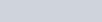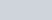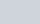Which term of the sequence 114, 109, 104,
Question:

Which term of the sequence 114, 109, 104, … is the first negative term?

Solution:

Here, A.P isSo, first term,Now,

Common difference (d) =$=109-114$

$=-5$

Now, we need to find the first negative term,

$a_{n}<0$

$114+(n-1)(-5)<0$

$114-5 n+5<0$

$119-5 n<0$

$5 n>119$

Further simplifying, we get,

$n>\frac{119}{5}$

$n>23 \frac{4}{5}$

$n \geq 24$ (as $n$ is a natural number)

Thus, $n=24$

Therefore, the first negative term is the $24^{\text {* term }}$ of the given A.P.